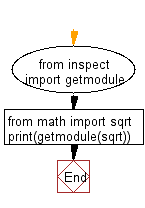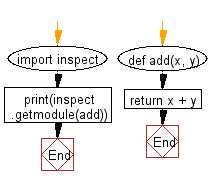﻿ Python: Get the actual module object for a given object - w3resource# Python: Get the actual module object for a given object

## Python Basic: Exercise-126 with Solution

Write a Python program to get the actual module object for a given object.

Sample Solution-1:-

Python Code:

``````from inspect import getmodule
from math import sqrt
print(getmodule(sqrt))
```
```

Sample Output:

```<module 'math' (built-in)>
```

Flowchart:Sample Solution-2:-

Python Code:

``````import inspect
return x + y
```
```

Sample Output:

```<module '__main__' from '/tmp/sessions/5ced515afe46d808/main.py'>
```

Flowchart:Python Code Editor:

Have another way to solve this solution? Contribute your code (and comments) through Disqus.

What is the difficulty level of this exercise?

Test your Python skills with w3resource's quiz

﻿

## Python: Tips of the Day

Try-catch-else construct:

```try:
foo()
except Exception:
print("Exception occured")
else:
print("Exception didnt occur")
finally:
print("Always gets here")
```